Thread: Eric Dollard View Single Postt-rexSenior Member Join Date: Aug 2011 Location: In the bushes Posts: 422
Law of Electro-Magnetic Induction, Twelve. (4 of 4)

Where it is,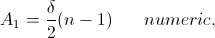A1 being the Lower Sideband Amplitude, and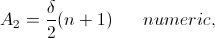A2 being the Upper Sideband Amplitude. Also,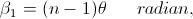The Lower Sideband Time Angle,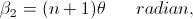The Upper Sideband Time Angle.

Note here that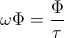Dimensionally establishes the volt.

Hence a pair of new Frequencies are generated by the synchronous parameter variation of Inductance, these given by the relations,

Lower Sideband Frequency,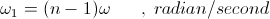Upper Sideband Frequency,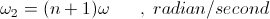Where,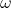, is the Carrier Frequency, of current variation via the A.C. cycle of the external current source.

Hence three alternating electric waves exist in the process of synchronous parameter variation, the values are given in Table 1,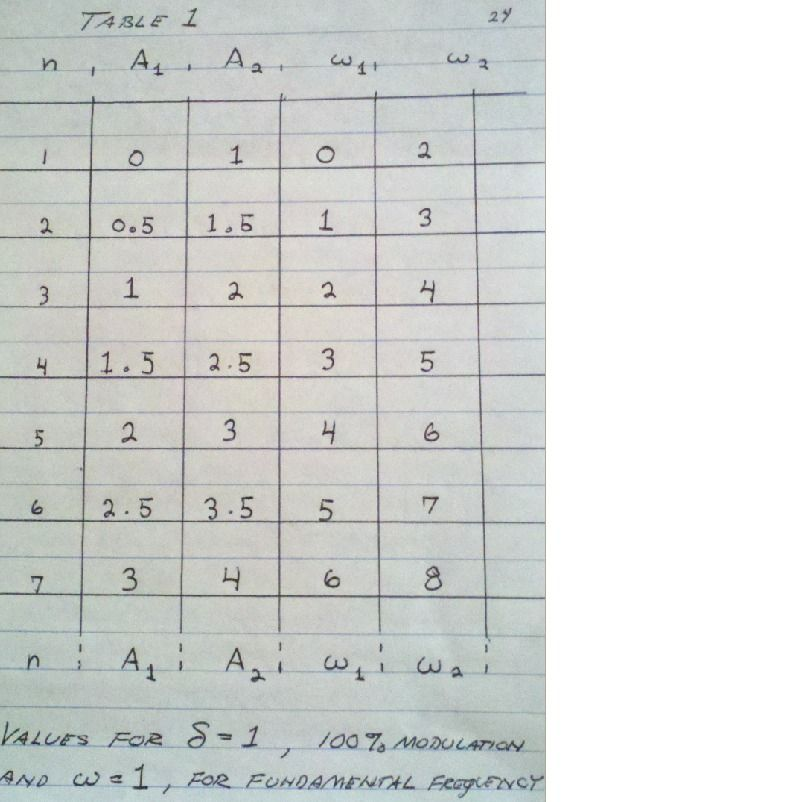Two particular harmonic modulating frequencies are of interest, the second harmonic and the fourth harmonic. For the condition of second harmonic modulation the frequency of the Lower Sideband is equal to the carrier frequency, these two combine in a resultant wave at the carrier frequency. The Upper Sideband is the third harmonic of the carrier frequency and super-imposed upon it creates a regular harmonic waveform. For the condition of fourth harmonic modulation the Lower Sideband gives the Third Harmonic of the carrier wave and the Upper Sideband gives the Fifth Harmonic of the carrier frequency. The odd order series, one, three, five exists here and again super-impose upon each other producing a regular harmonic waveform. All other harmonic modulating frequencies, three, five, etc, give rise to an irregular sequence and thus produce irregular harmonic waveforms. The depth of modulation as well as the Angle of Hysteresis both have a considerable effect on the resulting waveshape. This can give rise to very complex waveforms. It is to be noted that for large depth of modulation, and high orders of modulating harmonics, that the resulting E.M.F. can greatly exceed the E.M.F. of the reactance coil in reaction to the carrier frequency of the external A.C. current source. These processes are worthy of Experimental Research.

(9) Steinmetz does not develop this subject much further. The equations for parameter variation in stationary reactance coils, article 236, are dimensionally invalid,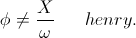A weber is not an ohm-second per radian, it is rightly given as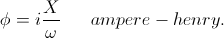The equations for harmonic summation are not clear and something seems not right. No in depth analysis exists of Resistance parameter variation on a theoretical level, everything is reduced to effective values. E.M.F. is not equated to a co-responding current in many cases making the study of Power Flow difficult in the case of Inductance parameter variation. It is noteworthy in this chapter that Steinmetz gives experimental verification of his parameter variation expressions. It is this feature of Steinmetz's work that makes it of value.

(10) Herewith closes this series of writings, The Law of Electro-Magnetic Induction. Three principle conditions for the development of electro-motive force have been presented,

Constant Magnetism,

Constant Current,

Constant Inductance.

While the condition of Constant Magnetism, the Motor-Generator, and the condition of Constant Inductance, the Reactance Coil, are well known engineering realities, it is the special condition of Constant Current that awaits further analysis and experimentation. In this particular condition of E.M.F. development the Law of Energy Continuity may be in need of re-definition. Here the Law of Energy Perpetuity, the holy dictum of modernistic physics, may possibly be invalidated.

73 DE N6KPH SK.....
__________________
SUPPORT ERIC DOLLARD'S WORK AT EPD LABORATORIES, INC.

Purchase Eric Dollard's Books & Videos - 70% of the sale goes to Eric and EPD Laboratories: Eric Dollard Books & Videos
Donate by Paypal: Donate to EPD Laboratories